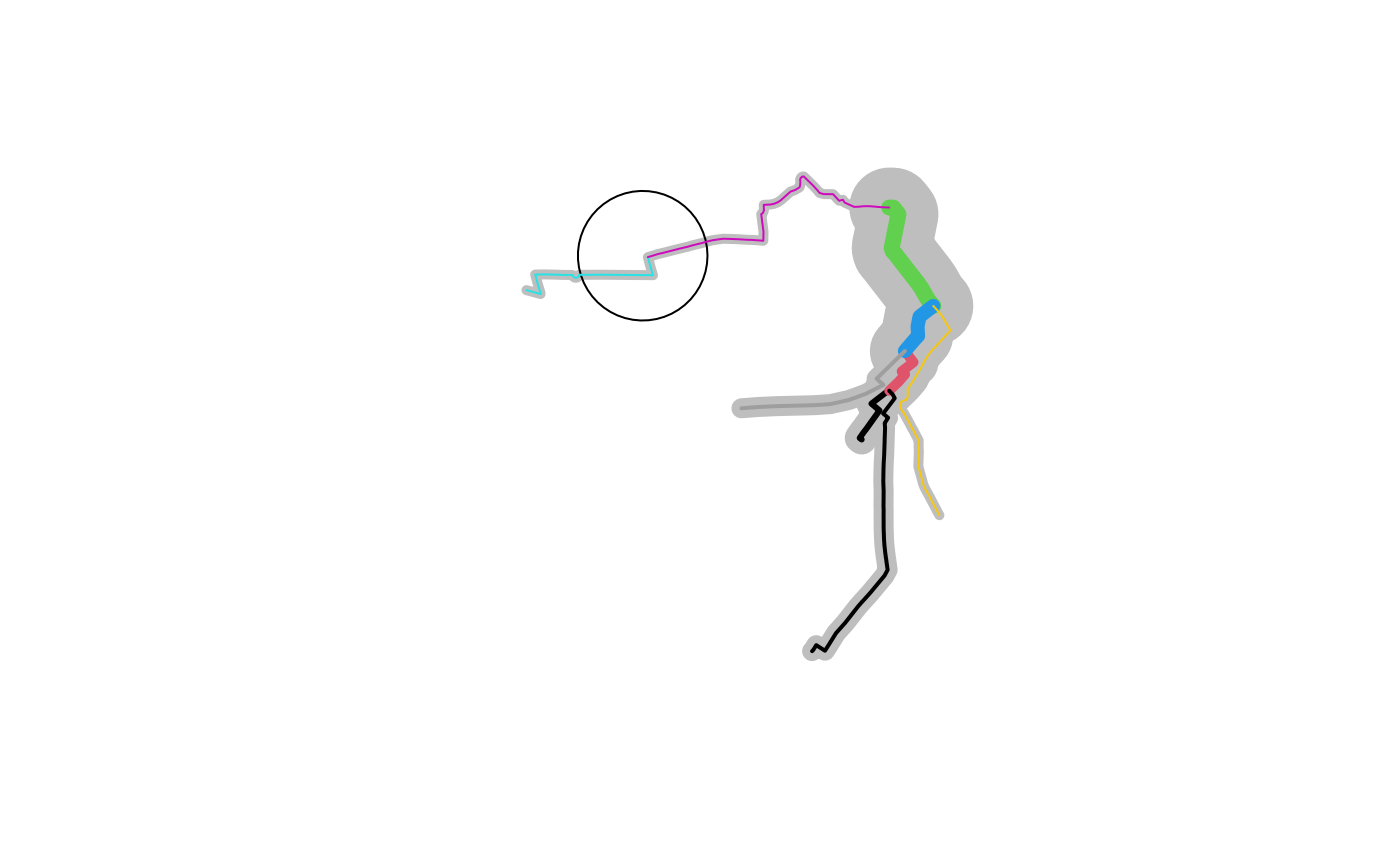Add a node to route network

## Usage

``rnet_add_node(rnet, p)``

## Arguments

rnet

A route network of the type generated by `overline()`

p

A point represented by an `sf` object the will split the `route`

## Examples

``````sample_routes <- routes_fast_sf[2:6, NULL]
sample_routes\$value <- rep(1:3, length.out = 5)
rnet <- overline2(sample_routes, attrib = "value")
p <- sf::st_sfc(sf::st_point(c(-1.540, 53.826)), crs = sf::st_crs(rnet))
r_split <- route_split(rnet, p)
plot(rnet\$geometry, lwd = rnet\$value * 5, col = "grey")
plot(p, cex = 9, add = TRUE)
plot(r_split, col = 1:nrow(r_split), add = TRUE, lwd = r_split\$value)``````